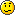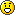[phpBB Debug] PHP Warning: in file [ROOT]/phpbb/session.php on line 580: sizeof(): Parameter must be an array or an object that implements Countable
[phpBB Debug] PHP Warning: in file [ROOT]/phpbb/session.php on line 636: sizeof(): Parameter must be an array or an object that implements Countable
[phpBB Debug] PHP Warning: in file [ROOT]/includes/functions.php on line 4511: Cannot modify header information - headers already sent by (output started at [ROOT]/includes/functions.php:3257)
[phpBB Debug] PHP Warning: in file [ROOT]/includes/functions.php on line 4511: Cannot modify header information - headers already sent by (output started at [ROOT]/includes/functions.php:3257)
[phpBB Debug] PHP Warning: in file [ROOT]/includes/functions.php on line 4511: Cannot modify header information - headers already sent by (output started at [ROOT]/includes/functions.php:3257)
snarescience.com • Schoolwork Help Thread - Page 2
Page 2 of 2

Posted: Sun Nov 28, 2010 8:33 pm
tommyservo7 wrote:
noname wrote: 2x+2z=2
5x+3y=4
3y-4z=4
The textbook says the solution should be (-4,8,5). How do you get this answer? I know it's something really simple, but I can't remember how to do it.
I tried. Looks like it requires a matrix.

EDIT: This nifty little thing works excellently. It will teach you how to do it.
Thanks for the help. If this is the way to go, thank you, but I don't this is how it was taught in class. If anyone else has an alternative method, please let me know.

Posted: Sun Nov 28, 2010 8:51 pm
noname wrote: 2x+2z=2
5x+3y=4
3y-4z=4
The textbook says the solution should be (-4,8,5). How do you get this answer? I know it's something really simple, but I can't remember how to do it.
2x+2z=2
5x+3y=4
3y-4z=4
It's a system of equations.

The first equation (solved for z):
z=1-x

Substitute this statement into the third equation:
3y-4(1-x)=4
3y-4+4x=4
3y+4x=8

Now you have a simple system of two variables. Solve with the second equation (equation addition and subtraction):
3y+4x=8
3y+5x=4
-x=4
x=-4

Now substitute this x-value into the first equation:

2(-4)+2z=2
-8+2z=2
2z=10
z=5

You may choose to use either the x-value or the z-value to solve for y. Let us use the z-value for variety:
3y-4(5)=4
3y-20=4
3y=24
y=8

Putting our three results into an ordered triple:
(x,y,z)
(-4,8,5)

Posted: Sun Nov 28, 2010 9:06 pm
AWA wrote:
noname wrote: 2x+2z=2
5x+3y=4
3y-4z=4
The textbook says the solution should be (-4,8,5). How do you get this answer? I know it's something really simple, but I can't remember how to do it.
2x+2z=2
5x+3y=4
3y-4z=4
It's a system of equations.

The first equation (solved for z):
z=1-x

Substitute this statement into the third equation:
3y-4(1-x)=4
3y-4+4x=4
3y+4x=8

Now you have a simple system of two variables. Solve with the second equation (equation addition and subtraction):
3y+4x=8
3y+5x=4
-x=4
x=-4

Now substitute this x-value into the first equation:

2(-4)+2z=2
-8+2z=2
2z=10
z=5

You may choose to use either the x-value or the z-value to solve for y. Let us use the z-value for variety:
3y-4(5)=4
3y-20=4
3y=24
y=8

Putting our three results into an ordered triple:
(x,y,z)
(-4,8,5)

That's more like what we learned in class. Thanks to both of you for your help.

Posted: Fri Feb 04, 2011 3:57 am
My homework will be due before anyone sees this, but here's a couple questions.

This one's multiple choice:
The average velocity of an object for a given motion points to the right. When is it possible for the instantaneous velocity of the object to point to the left? (Select all that apply.)
-at the beginning of the motion
-at some point in the middle of the motion
-at the end of the motion

I just 100% don't get this question and so I got it wrong.

Also this:

You drive in a straight line at 18.0 m/s for 12.0 miles, then at 30.0 m/s for another 12.0 miles.
(a) How does your average speed compare with 24.0 m/s?
speedav < 24.0 m/s
speedav > 24.0 m/s
speedav = 24.0 m/s
Explain.

I originally was like "oh I'm traveling different speeds over the same distance so I can just add those and take the average of that" which ended up being wrong. Later on in the question you calculate the average velocity and I realized that since you are traveling the same distance with different speeds, the time in which you travel those distances is different so you can't really take a shortcut there and you have to actually calculate out the distance traveled over time. Right? Does that make sense?Posted: Fri Feb 04, 2011 11:30 am
waffley wrote:My homework will be due before anyone sees this, but here's a couple questions.

This one's multiple choice:
The average velocity of an object for a given motion points to the right. When is it possible for the instantaneous velocity of the object to point to the left? (Select all that apply.)
-at the beginning of the motion
-at some point in the middle of the motion
-at the end of the motion

I just 100% don't get this question and so I got it wrong.

Also this:

You drive in a straight line at 18.0 m/s for 12.0 miles, then at 30.0 m/s for another 12.0 miles.
(a) How does your average speed compare with 24.0 m/s?
speedav < 24.0 m/s
speedav > 24.0 m/s
speedav = 24.0 m/s
Explain.

I originally was like "oh I'm traveling different speeds over the same distance so I can just add those and take the average of that" which ended up being wrong. Later on in the question you calculate the average velocity and I realized that since you are traveling the same distance with different speeds, the time in which you travel those distances is different so you can't really take a shortcut there and you have to actually calculate out the distance traveled over time. Right? Does that make sense?I didn't quite understand the first one, but I do get the second one.

18 m/s = 12/t1, 12/18 s = t1
30 m/s = 12/t2, 12/30 s = t2

Average velocity = dd/dt, or 24/t1+t2

Because t1+t2 > 1 (12/18 is 2/3, 12/30 > 10/30 = 1/3), we know that the average velocity that you traveled will be < 24 m/s.

Posting this in here because everyone should know about this awesome guy who should probably be a tenured professor at some awesome university.